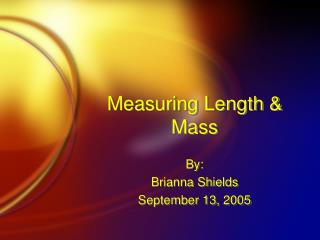DownloadDownload PresentationMeasuring Length & Mass

# Measuring Length & Mass

Télécharger la présentation## Measuring Length & Mass

- - - - - - - - - - - - - - - - - - - - - - - - - - - E N D - - - - - - - - - - - - - - - - - - - - - - - - - - -
##### Presentation Transcript

1. Measuring Length & Mass By: Brianna Shields September 13, 2005

2. DO NOW • 1. What tool is used to measure length? • 2. What tool is used to measure mass? • 3. What tool is used to measure temperature?

3. Definition Distance between two points Length

4. Tool Ruler or meterstick Length

5. Label units Centimeter (cm) Length

6. Tips Line object up at zero mark Measure with decimals (no fractions) Be accurate Label your answer Length

7. Example • What is the length of the object at left?

8. Example • What is the length of the object at left?

9. Definition Amount of matter in an object Mass

10. Tool Triple beam balance Mass

11. Label units Grams (g) Mass

12. Using a tbb 1. Zero the balance (all masses left)

13. Using a tbb 2. Move 100 g mass first- until balance tips, then slide mass one spot left

14. Using a tbb 3. Move 10 g mass next until balance tips, then slide mass one spot left

15. Using a tbb 4. Move 1 g mass last until balance tips, then slide mass one spot left

16. Using a tbb 5. Add up all three masses to find the total mass

17. Tips Be accurate Use decimals, no fractions Label your answer correctly Mass

18. Example • What is the mass of the object to the left?

19. Example • What is the mass of the object to the left?

20. Practice • Work with your lab partner • Complete the length and mass practice questions in your handout • We will go over the correct answers at the end of the period